##### Are Near Earth Objects the Key to Optimization Theory

Richard A. Formato

Journal of Advances in Mathematics and Computer Science, Page 341-351
DOI: 10.9734/BJMCS/2013/3400

This note suggests that near earth objects and Central Force Optimization have something in common, that NEO theory may hold the key to solving some vexing problems in deterministic optimization: local trapping and proof of convergence. CFO analogizes Newton’s laws to locate the global maxima of a function. The NEO-CFO nexus is the striking similarity between CFO’s Davg and an NEO’s ΔV curves. Both exhibit oscillatory plateau-like regions connected by jumps, suggesting that CFO’s metaphorical “gravity” indeed behaves like real gravity, thereby connecting NEOs and CFO and being the basis for speculating that NEO theory may address difficult issues in optimization.

##### A Homotopy Continuation Approach for Testing a Basic Analog Circuit

H. Vazquez-Leal, A. Sarmiento-Reyes, U. Filobello-Nino, Y. Khan, A. L. Herrera-May, R. Castaneda-Sheissa, V. M. Jimenez-Fernandez, M. Vargas-Dorame, J. Sanchez-Orea

Journal of Advances in Mathematics and Computer Science, Page 226-240
DOI: 10.9734/BJMCS/2013/3116

The increase of complexity on integrated circuits has also raised the demand for new testing methodologies capable to detect functional failures within circuits before they reach the market. Hence, this work proposes to explore the use of homotopy as a tool for testing a basic analog circuit. The homotopy path is influenced by nonlinearities from the equilibrium equation of the circuit; this situation can be used to infer faults by detecting changes on the homotopy path. The concept was explored using numerical simulation of a simple test circuit; then comparing results for the circuit with and without faults, obtaining modifications on the homotopy path like: the final point, number of iterations, and the number of turning points.

##### Pseudorandomness in Central Force Optimization

Richard A. Formato

Journal of Advances in Mathematics and Computer Science, Page 241-264
DOI: 10.9734/BJMCS/2013/3381

Central Force Optimization is a deterministic metaheuristic for an evolutionary algorithm that searches a decision space by flying probes whose trajectories are computed using a gravitational metaphor. CFO benefits from the inclusion of a pseudorandom component (a numerical sequence that is precisely known by specification or calculation but otherwise arbitrary). The essential requirement is that the sequence is uncorrelated with the decision space topology, so that its effect is to pseudorandomly distribute probes throughout the landscape. While this process may appear to be similar to the randomness in an inherently stochastic algorithm, it is in fact fundamentally different because CFO remains deterministic at every step. Three pseudorandom methods are discussed (initial probe distribution, repositioning factor, and decision space adaptation). A sample problem is presented in detail and summary data included for a 23-function benchmark suite. CFO’s performance is quite good compared to other highly developed, state-of-the-art algorithms.

##### Bounds for the Convex Combination of the First Seiffert and Logarithmic Means in Terms of Generalized Heronian Mean

Journal of Advances in Mathematics and Computer Science, Page 265-274
DOI: 10.9734/BJMCS/2013/3049

In this paper, the bounds for convex combination of the ﬁrst Seiffert and Logarithmic means by
general Heronian meanare proved, where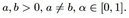The left bound is the best possible.

##### A New Six Dimensional Representation of the Braid Group on Three Strands and its Irreducibility and Unitarizability

Journal of Advances in Mathematics and Computer Science, Page 275-280
DOI: 10.9734/BJMCS/2013/3141

We consider the braid group on three strands, B3and construct a complex valued representation of it with degree 6, namely,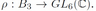First, we show that this representation is irreducible and not equivalent to either Burau or Krammer’s representations. Second, we prove that the representation is unitary relative to an invertible hermitian matrix.

##### Three Solutions for a Navier Boundary Value System Involving the (p(x); q(x))-Biharmonic Operator

Honghui Yin, Zuodong Yang

Journal of Advances in Mathematics and Computer Science, Page 281-290
DOI: 10.9734/BJMCS/2013/3166

The existence of at least three weak solutions is established for a class of quasilinear elliptic systems involving the (p(x); q(x))-biharmonic operators with Navier boundary value conditions. The technical approach is mainly based on a three critical points theorem due to Ricceri .

##### Several Asymptotic Products of Particular Distributions

C.K. Li

Journal of Advances in Mathematics and Computer Science, Page 291-303
DOI: 10.9734/BJMCS/2013/2721

The problem of deﬁning products of distributions is a difﬁcult and not completely understood problem, studied from several points of views since Schwartz established the theory of distributions around 1950. Many ﬁelds, such as wave propagation or quantum mechanics, require such multiplications. The product of an inﬁnitely differentiable function (x) and distribution 4(x) inRn is well deﬁned by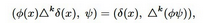sinceUsing an induction, we derive an interesting formula for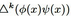and hence we are able to write out an explicit expression of the product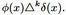In particular, we imply the product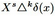with a few applications in further simplifying existing distributional products. Furthermore, we obtain an asymptotic expression for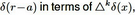which isequivalent to the well-known Pizzetti’s formula. Several asymptotic products including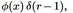as wellasthemore generalizedare calculated and presented as inﬁnitely series.

##### The Existence and Nonexistence of Entire Positive Radial Solutions of Quasilinear Elliptic Systems with Gradient Term

Hongxia Qin, Zuodong Yang

Journal of Advances in Mathematics and Computer Science, Page 304-314
DOI: 10.9734/BJMCS/2013/2498

We study the existence and nonexistence of entire positive solutions for quasilinear elliptic systemon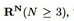where nonlinearities f and g are positive and continuous, the potentials a and b are continuous, c-positive and satisfy appropriate growth conditions at inﬁnity. We have that entire large positive solutions fail to exist if f and g are sublinear and a and b have fast decay at inﬁnity, while if f and g satisfy some growth conditions at inﬁnity, and a; b are of slow decay or fast decay at inﬁnity, then the system has inﬁnitely many entire solutions, which are large or bounded.

##### Momentum-Entire Wavelets with Discrete Rotational Symmetries in 2D

Guy Battle

Journal of Advances in Mathematics and Computer Science, Page 315-329
DOI: 10.9734/BJMCS/2013/2481

We introduce wavelet bases consistent with the eigenspaces of the action of rotation by the angle 2π / N in dimension d = 2. Our particular construction yields wavelets that are momentrum-entire (a property weaker than the compact support property). The orthogonality of wavelets in a given eigenspace is based on an inner product that depends on the eigenspace, while the eigenspaces themselves form a super-orthogonal system over a certain family of Hilbert spaces. (We describe this notion in the Introduction.) The existence of a gradient-orthonormal basis of momentum-entire wavelets is an issue that remains open.

##### Oscillation Criteria for the Solutions of Higher Order Functional Dierence Equations of Neutral type

Radhanath Rath, Ajit kumar Bhuyan, B.L.S. Barik

Journal of Advances in Mathematics and Computer Science, Page 330-340
DOI: 10.9734/BJMCS/2013/3481

In this paper, necessary and sufficient conditions are obtained so that the neutral functional difference equation $$\Delta^{m}\big(y_n-y_{\tau(n)}\big) + q_nG(y_{\sigma(n)})=f_n,\quad n\geq n_0,$$ admits a positive bounded solution, where $$m \geq 1$$ is an odd integer, $$\Delta$$ is the forward difference operator given by $$\Delta y_n = y_{n+1}-y_n$$; $$\{f_n\}$$, $$\{q_n\}$$, are sequences of real numbers with $$q_n \geq 0$$, $$G \in C(\mathbb{R},\mathbb{R}).$$ The results of this paper improve and extend some recent work [6,15].

##### Lossless Transmission Lines Terminated by L-Load in Series Connected to Parallel Connected GL-Loads

Vasil G. Angelov

Journal of Advances in Mathematics and Computer Science, Page 352-389
DOI: 10.9734/BJMCS/2013/3622

The paper analyses transmission lines terminated by nonlinear loads situated as follows: GC-loads are connected parallel and L-load is connected in series to them (Fig. 1). First, we formulate boundary conditions for a lossless transmission line system on the basis of Kirchhoff’s law. Then, we reduce the mixed problem for the system in question to initial value problem for a neutral system on the boundary. We introduce an operator in a suitable function space whose fixed point is a periodic solution of the neutral system. The obtained conditions are easily verifiable. We demonstrate the advantages of our method in Numerical example.

##### Orthogonal Double Covers of Complete Bipartite Graphs by A Special Class of Disjoint Union of Path and A Complete Bipartite Graph

R. El-Shanawany, H. Shabana

Journal of Advances in Mathematics and Computer Science, Page 390-396
DOI: 10.9734/BJMCS/2013/3352

Let H be a graph on n vertices and let G be a collection of n subgraphs of H, one for each vertex, G is an orthogonal double cover (ODC) of H if every edge of H is contained in exactly two members of G and any two members share an edge whenever the corresponding vertices are adjacent in H and share no edges whenever the corresponding vertices are non-adjacent in H. In this paper, we are concerned with the symmetric starter vectors of the orthogonal double covers of the complete bipartite graphs and using this method to construct ODCs by the disjoint union of path and a complete bipartite graph. Here, we consider Pm the path on m vertices where 4 ≤ m ≤ 11:

##### Numerical Integration with Exponential Fitting Factor for Singularly Perturbed Two Point Boundary Value Problems

K. Madhu Latha, K. Phaneendra, Y. N. Reddy

Journal of Advances in Mathematics and Computer Science, Page 397-414
DOI: 10.9734/BJMCS/2013/3953

In this paper, we discuss the numerical integration with exponential fitting factor for singularly perturbed two-point boundary value problems. It is based on the fact that: the given SPTPBVP is replaced by an asymptotically equivalent delay differential equation. Then, numerical integration with exponential fitting factor is employed to obtain a tridiagonal system which is solved efficiently by Thomas algorithm. We discussed convergence analysis of the method. Model examples are solved and the numerical results are compared with exact solution.

##### Convergence Theorems on Generalized Strongly Successively Ø-pseudocontractive Mappings in the Intermediate Sense

G. A. Okeke, H. Olaoluwa

Journal of Advances in Mathematics and Computer Science, Page 415-424
DOI: 10.9734/BJMCS/2013/2430

We introduce a new class of nonlinear mappings, the class of generalized strongly successively Φ - pseudocontractive mappings in the intermediate sense and prove the convergence of Mann type iterative scheme to their fixed points. Our results improves and generalizes several other results in literature.

##### On Cyclic Orthogonal Double Covers of Circulant Graphs using Infinite Graph Classes

M. Higazy

Journal of Advances in Mathematics and Computer Science, Page 425-436
DOI: 10.9734/BJMCS/2013/4165

An orthogonal double cover (ODC) of a graph  is a collection  of  subgraphs of  such that every edge of  is contained in exactly two members of  and for any two members  and  in ,  is 1 if  and it is 0 if . An ODC  of  is cyclic (CODC) if the cyclic group of order  is a subgroup of the automorphism group of . In this paper, the CODCs of certain circulants with a specific regularity by certain infinite graph classes are concerned.

##### On Convergence and Stability of the Generalized Noor Iterations for a General Class of Operators

H. AKEWE, J. O. OLALERU

Journal of Advances in Mathematics and Computer Science, Page 437-447
DOI: 10.9734/BJMCS/2013/2724

In this paper, we establish some strong convergence and stability results of multistep iterative scheme for a general class of operators introduced by Bosede and Rhoades  in a Banach space. As corollaries, some convergence and stability results for the Noor, Ishikawa, Mann and Picard iterative schemes are also established. Our convergence results generalize and extend the results of Berinde , Bosede , Olaleru , Rafiq [21, 22] among others, while our stability results are extensions and generalizations of multitude of results in the literature, including the results of Berinde , Bosede and Rhoades , Imoru and Olatinwo  and Osilike .

##### Numerical Fractional Differentiation: Stability Estimate and Regularization

Ailin Qian, Guangfu Wang

Journal of Advances in Mathematics and Computer Science, Page 448-457
DOI: 10.9734/BJMCS/2013/3921

It is well known that the problem of fractional differentiation n is an ill-posed problem. So far there exists many approximation methods for solving this problem. In this paper we prove a stability estimate for a problem of fractional differentiation. Based on the obtained stability estimate, we present a Tikhonov regularization method and obtain the error estimate. According to the optimality theory of regularization, the error estimates are order optimal. Numerical experiment shows that the regularization works well.